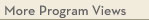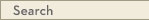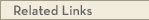# University Catalogs

Duluth campusDuluth Campus

# Mathematics Minor

Mathematics & Statistics
Swenson College of Science and Engineering
• Program Type: Undergraduate minor related to major
• Requirements for this program are current for Spring 2019
• Required credits in this minor: 23
The minor in mathematics is based on the completion of the traditional core of calculus, differential equations, and elementary linear algebra commonly required of undergraduate physical science, engineering, and mathematics degrees. Additional, more advanced, elective classes are required, as well. The minor in mathematics certifies a student's quantitative, problem-solving, and critical thinking skills.
Program Delivery
This program is available:
• via classroom (the majority of instruction is face-to-face)
Minor Requirements
Core Courses (14 cr)
Calculus I
MATH 1290 - Calculus for the Natural Sciences [LE CAT2, LOGIC & QR] (5.0 cr)
or MATH 1296 - Calculus I [LE CAT, LOGIC & QR] (5.0 cr)
Calculus II
MATH 1297 - Calculus II [LOGIC & QR] (5.0 cr)
Core Course
MATH 3280 - Differential Equations with Linear Algebra (4.0 cr)
Electives (9 cr)
Each course must be a minimum of 3 credits. MATH 3326 not allowed in electives. STAT 3411 OR STAT 3611 may be taken but both cannot be taken as required elective credit.
Take 3 or more course(s) totaling 9 or more credit(s) from the following:
· MATH 3xxx
· MATH 4xxx
· MATH 5xxx
· STAT 3xxx
· STAT 4xxx
· STAT 5xxxView college catalog(s): · Swenson College of Science and Engineering View checkpoint chart: · Mathematics Minor View PDF Version:Search Programs Search University CatalogsOne Stop for tuition, course registration, financial aid, academic calendars, and moreMATH 1290 - Calculus for the Natural Sciences (LE CAT2, LOGIC & QR)
 Credits: 5.0 [max 5.0] Course Equivalencies: 01420 - Math1290/1296/1596 Grading Basis: A-F or Aud Typically offered: Every Fall & Spring
Differential and integral calculus needed for modeling in earth and life sciences. Computational software. Not intended for students in mathematics, engineering, or physical sciences. prereq: Math ACT 27 or higher or a grade of at least C- in Math 1250 or department consent
MATH 1296 - Calculus I (LE CAT, LOGIC & QR)
 Credits: 5.0 [max 5.0] Course Equivalencies: 01420 Grading Basis: A-F or Aud Typically offered: Every Fall, Spring & Summer
First part of a standard introduction to calculus of functions of a single variable. Limits, continuity, derivatives, integrals, and their applications. prereq: Math ACT 27 or higher or a grade of at least C- in Math 1250 or department consent
MATH 1297 - Calculus II (LOGIC & QR)
 Credits: 5.0 [max 5.0] Course Equivalencies: 01421 Grading Basis: A-F or Aud Typically offered: Every Fall, Spring & Summer
Second part of a standard introduction to calculus. Vectors, applications of integrals, transcendental functions, series, and multivariable functions and partial derivatives. prereq: A grade of at least C- in 1290 or 1296 or 1596
MATH 3280 - Differential Equations with Linear Algebra
 Credits: 4.0 [max 4.0] Grading Basis: A-F or Aud Typically offered: Every Fall, Spring & Summer
First, second, and higher order equations; series methods; Laplace transforms; systems; software; modeling applications; introduction to vectors; matrix algebra, eigenvalues. prereq: A grade of at least C- in 1297 or 1597